Subscribe to INVBAT.COM -AI + CHATBOT
Get personal memory assistant and augmented intelligence on demand using many devices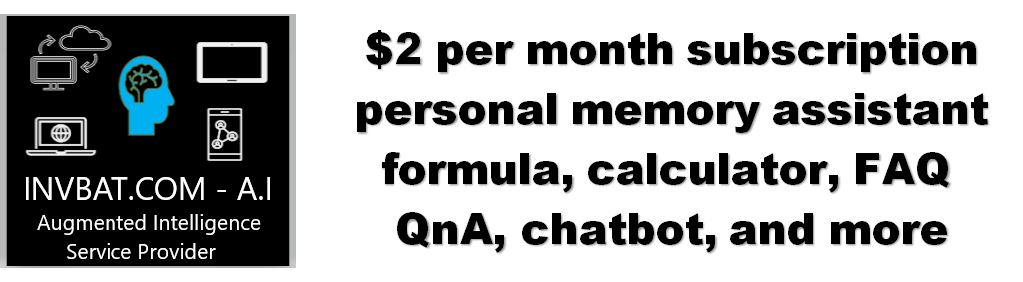How do you like the idea of NOT saying " Oh I forgot, let me get back to you. "INVBAT.COM - AI revolutionizing education and how to remember using augmented intelligence.

## ASK WHAT YOU NEED USING VOICE OR TEXT search pattern instruction

Try typing or saying I need the quadratic formula
Try typing or saying get me the quadratic formula
Try typing or saying show me the quadratic formula

Enter your search. Then click fewer click search button

If nothing is found probably because you are not a subscriber.
Try our subscription.

AI Natural Language Query makes one click search now possible

INVBAT.COM - A.I. + CHATBOT voice search for formula , calculator, reviewer, work procedure and frequently asked questions (FAQ). It is useful immediately and on demand using your smartphone, notebook, tablet, laptop or desktop computer. Helping you to learn faster and 98% never forget.

INVBAT.COM - AI + CHATBOT is a personalized natural language search and information retrieval augmented intelligence service provider. We deliver immediate usefulness at affordable cost to our subscriber such as students, teachers, parents, and employees to help them remember what they stored in the cloud in one or fewer click using their smartphone, tablet, laptop, desktop computer, and smart tv.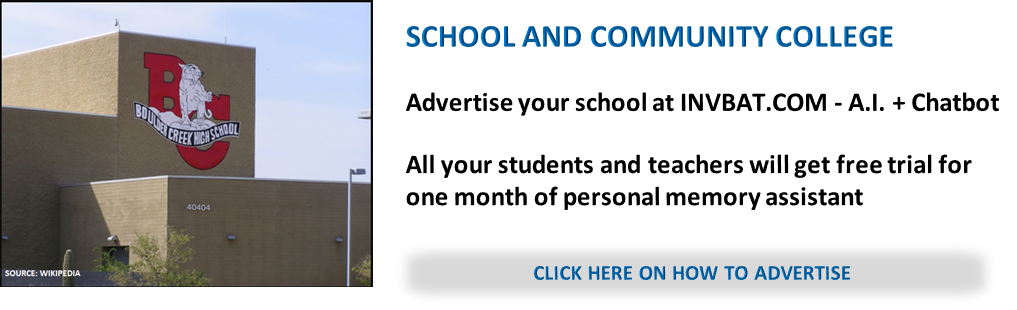Additional bonus if your school , community college, and university advertise, all your students and teachers will get free personal memory assistant chatbot for one month.

Advertise on this webpage. Use PayPal

# BECAUSE MOST OF US FORGETINSTRUCTION ON HOW TO USE: Enter 2 in input box X 2 and 1 in input box X then - 6. You will get factors in simplified form

X2 + X + = 0

If the answer is NaN (Not a Number). It means the answer is imaginary number . See answer below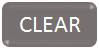The initial pattern for solving this type of problem is remembering the formula. Next mastering how to use your personal online calculator. Now you have your tool for computational thinking. You can do quick analysis. For example you can adjust 2 to 8, 1 to -1 and -6 to -11 . Tip do not enter comma symbol because you will get an error message of NaN, meaning Not a Number data entry.

( X + ) ( X + ) = 0 ANSWER : FACTORS OF EQUATION

First root: X1 = If answer is NaN, it means the answer is imaginary number.

X1 = b + i The answer is imaginary number.

Second root: X2 = If answer is NaN, it means the answer is imaginary number.

X2 = b - i The answer is imaginary number.

X1 = ( b + Square Root ( b2 - 4 a * c ) ) / 2 * a

X1 = ( b + Square Root ( ) ) / =

X2 = ( b - Square Root ( b2 - 4 a * c ) ) / 2 * a

X2 = ( b - Square Root ( ) ) / =

APPLICATION OF LESSON LEARNED:

Example (X + 2)(X - 1.5) = 0 is equivalent to quadratic equation x2 + 0.5 x - 3 = 0

(X + 2)(X - 1.5) = 0 means when the value of y is set to zero (0) you can solve the value of x as shown by the graph below. (X + 2)(X - 1.5) = y(0)
y(0) is interpreted as , you set the value of y equal to zero then solve for the value x. Then you will get a pair coordinates (-2, 0 ) and (1.5, 0 )

y(1) is interpreted as, you set the value of x equal to 1 then solve for the value y. The answer is y(1) = -1.5 . Now you will have a new coordinate point (1, -1.5) and (-1, -1.5).

y(x) can be interpreted as the generalized quadratic equation or parabolic equation meaning you can assigned any real number value for x-coordinate and you can predict or expect a corresponding y-coordinate value.

y(x) is sometimes written as f(x) meaning the output value f(x) is a function of any input value of x. The lesson you learned about x-coordinate and y-coordinate is very important concept that you needed in real work application like Pi display dashboard, computer animation and factory automation.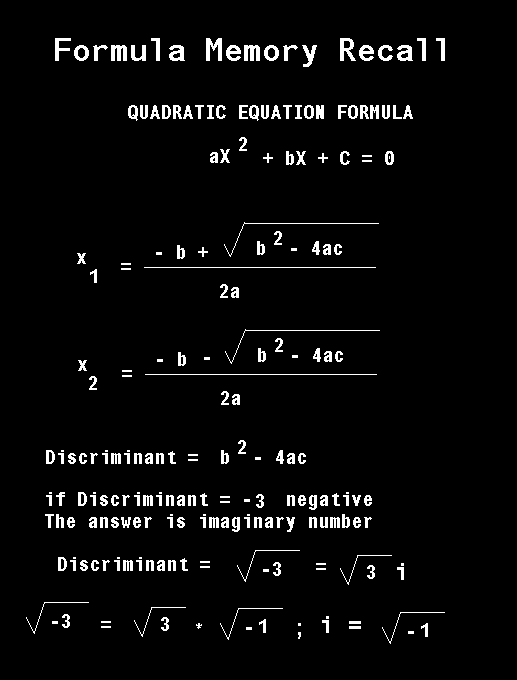Below is the solution using graphing method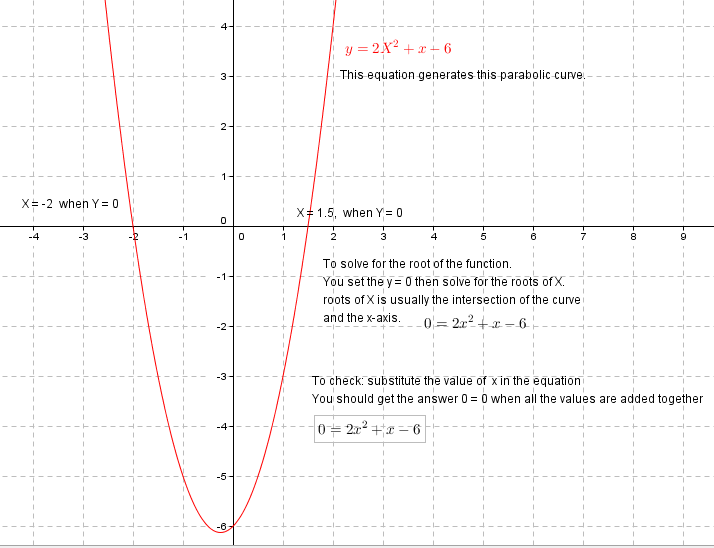Graph created using GeoGebra

Click the link to solve using Wolfram AlphaHow do you know that learning happen in student's brain or knowledge was transferred to student's brain?

If the student can remember the procedural instruction how to solve the problem by using his memory only.

INVBAT.COM - A.I. + CHATBOT is very helpful for this kind of knowledge retrieval task because cloud technology have high speed , have large memory capacity and have fast computing power compared to human brain. Therefore human brain need Augmented Intelligence (Au.I.) to manage and control future advancement in Artificial Intelligence (Ar. I ) . Students, teachers, and employees today are busy they need on demand help to quickly review the procedural instruction. They also need automatic calculator for fast solution. By subscribing to INVBAT.COM - A.I. CHATBOT they will get on demand help using their smartphone, tablet, notebook, laptop, desktop, school smartboard, and even company smart T.V. in conference room.

INVBAT.COM -A.I. CHATBOT is solving previously impossible efficiency and convenience question. Example of impossible question 10 years ago, Can you combine formula and calculator make it available for immediate use using many devices? That is an impossible question 10 years ago ask by the founder of INVBAT.COM. Today that question is now considered as solved problem. You can help to grow the database of combined formula and calculator by subscribing or advertising to INVBAT.COM - A.I. + CHATBOT

CHATBOT ID number available for subscription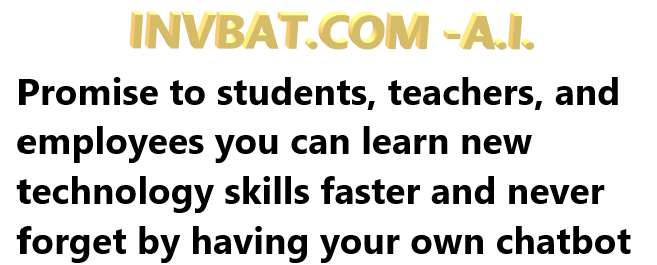## INVBAT.COM - A.I. a disruptive innovation in computing and web search technology.

INVBAT.COM - A.I. is a disruptive innovation in computing and web search technology. For example scientific calculator help us speed up calculation but we still need to remember accurately the formula and the correct sequence of data entry. Here comes the disruptive innovation from INVBAT.COM-A.I. , today the problem of remembering formula and the correct sequence of data entry is now solved by combining formula and calculation and make it on demand using smartphone, tablet, notebook, Chromebook, laptop, desktop, school smartboard and company big screen tv in conference room with internet connection.

For web search , INVBAT.COM-A.I, is demonstrating that you can type text or use voice to text A.I. to search the web and get direct answer in one or two clicks. You don't need to waste your time looking from million of search results.